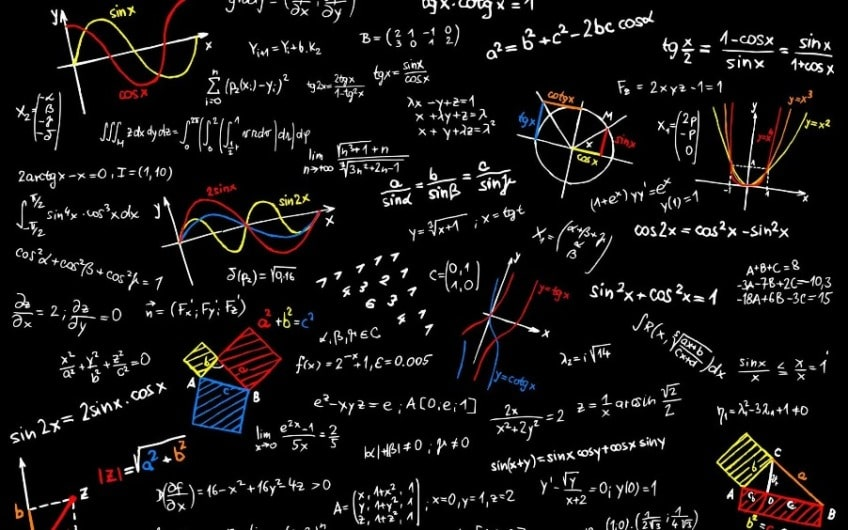November 29, 2023In Mathematics, fractions are represented as a numerical value, which defines a part of a whole. They have numerator and denominator and based on these two terms, fractions can be classified into three types. They are proper fractions, improper fractions and mixed fractions.

There are several ways we use fractions unknowingly while performing our day to day activities. From expressing exam scores to dividing a cake into equal slices, fractions are used everywhere. It is fair to say that the world as we know it now would not exist without fractions.

Let’s learn about one of the most important fraction type i.e., Proper Fractions and how can one convert Fraction to Percentage to get a value that is easier to remember than a fraction.

## What is a Proper Fraction?

A proper fraction is one that has a smaller numerator than its denominator. It is called a proper fraction because it adheres to the definition of a fraction and the need for a fraction. To better understand this, consider how they differ from improper fractions.

Improper fractions have a denominator that is less than the numerator. If we recall the concept of fractions, we will see that if the denominator is less than the numerator in a fraction, the total value of the fraction will be larger than one, which complicates matters. It should be noted that if a fraction is bigger than one, it can be divided into a whole number and a fractional part.

For example, 5/2 indicates two and a half, which can be represented as 21/2 and is known as a mixed number. This means that 5/2 is made up of two whole parts and half of one whole portion. As a result, 5/2 is considered improper because it may be expressed as both a whole number and a proper fraction (1/2).

### Examples of Proper Fractions

• A proper fraction is four out of six equal pizza slices, which can be represented as 4/6 or 2/3, where the numerator is less than the denominator.
• A valid fraction is 80 out of 100 marks in an exam because the number of marks scored is fewer than the total number of marks. The fraction can be represented as 80/100, and its simplified version is 4/5.
• A proper fraction can be 3 out of 20 students in a group, where the number of students in the statement (3) is less than the total number of students in the group (20). The fraction is written as 3/20.
• A proper fraction is 110 pages out of 530 in a book, which can be expressed as 110/530 or 11/53. The number of pages under consideration in this case is less than the total number of pages in the book, implying that the numerator is less than the denominator.

## How to Convert Fraction to Percent?

To convert a fraction to a percentage, simply multiply it by 100 and reduce it to percent. Here is an example to help you learn how to convert a fraction to a percentage. Let’s discuss the steps to convert a fraction to a percentage:

• Convert the fraction to decimal form.
• To obtain a percent value, multiply the resulting decimal number by 100.

To understand the concept of converting fraction to percentage, consider that if a class has 42 students – among them 21 are male. Now, what is the percentage of male students?

It is 21 out of 42. To simplify: 21/42 = 0.5 or about 50%.